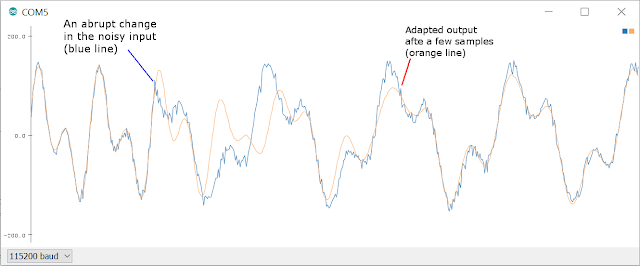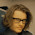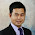## Sunday, April 17, 2016

1. Fourier Linear Combiner (FLC)
2. Weighted-frequency Fourier Linear Combiner (WFLC)
3. Bandlimited Multiple Fourier Linear Combiner (BMFLC)

FLC estimates the quasiperiodic signal of known frequency by using least mean square (LMS) algorithm to adapt the amplitude and phase of a reference signal. WFLC is an extension of FLC which can adapt to a periodic signal of unknown frequency and amplitude. Consequently, WFLC also adapts to time-varying reference signal frequencies while FLC can only estimate a signal with fixed and known frequency. To eliminate the time lag which is not desirable in real-time application, a method using combination of WFLC-FLC has been proposed. One limitation of WFLC is its inability to extract a periodic or quasi-periodic signal containing more than one dominant frequency. To overcome that, BMFLC approach tracks a predetermined band of multiple dominant frequencies based on the prior knowledge of the desired signal. The adaption process is achieved using LMS optimization similar to WFLC and FLC. As the frequency components in BMFLC are constant, analytical ouble integration can be employed to obtain the displacement from acceleration. Due to this reason, it becomes an ideal choice for applications where data is sensed with accelerometers.

### Setup

Arduino zero pro is used for the experiment. The code are written in C so that it can be ported to other platforms easily. Firstly, a reference signal is generated which is superimposed with noise. The generated signal is filtered with adaptive filter. The filtered output is compared with the reference signal by plotting them on serial plotter. The latest version Arduino IDE has not only Serial Monitor but it also has Serial Plotter. Therefore, it is easy and convenient to plot and see them serial plotter how an adaptive filter adapts to its input signal.

### FLC

A periodic signal with fundamental frequency, $$f_0$$ can be modeled and estimated in real-time with Fourier Linear Combiner (FLC) [Vaz, 1994]. Least mean square (LMS) algorithm is used to adapt fourier components in FLC and its architecture is shown below.

Fourier linear combiner (FLC) is represented with a summation of sine and cosine components as follow. $$y_k=\sum^n_{r=1}a_{rk}\sin(r\omega_0 k)+b_{rk}\cos(r\omega_0 k)$$ where k in sampling instant, $$y_k$$ is the estimated signal, and $$a_{rk}$$ and $$b_{rk}$$ are adaptive weights for their respective harmonic frequency $$r\omega_0$$.

If $$x_k$$ is reference input vector and $$w_k$$ is weight vector, then the estimated signal $$y_k$$ can be represented as in the following equation. $$\begin{equation} \mathbf{x}_k=\begin{bmatrix} \begin{bmatrix} \sin(\omega_{0} k) & \sin(2\omega_{0} k) & \dots & \sin(n\omega_{0} k) \end{bmatrix}^T\\ \begin{bmatrix} \cos(\omega_{0} k) & \cos(2\omega_{0} k) & \dots & \cos(n\omega_{0} k) \end{bmatrix}^T\\ \end{bmatrix} \end{equation}$$ $$\begin{equation} \mathbf{w}_k=\begin{bmatrix} \begin{bmatrix} a_{1k} & a_{2k} & \dots & a_{nk} \end{bmatrix}^T\\ \begin{bmatrix} b_{1k} & b_{2k} & \dots & b_{nk} \end{bmatrix}^T\\ \end{bmatrix} \end{equation}$$ $$\begin{equation} y_k=\mathbf{w}_k^T \mathbf{x}_k \end{equation}$$ The weights of FLC are updated as described in the following equations. $$\begin{equation} \epsilon_k=s_k-y_k \end{equation}$$ $$\begin{equation} \mathbf{w}_{k+1}=\mathbf{w}_k +2 \mu \mathbf{x}_k \epsilon_k \end{equation}$$

where $$x_k$$ is reference input vector, $$s_k$$ is reference signal, $$\epsilon_k$$ is error term, and $$\mu$$ is adaptive gain parameter. The smaller the value of $$\mu$$, the slower the adaptation rate. If the value of $$\mu$$ is too big, the filter falis to adapt and it becomes unstable. Time constant for convergence is $$\tau = \frac{1}{2\mu}$$. If the DC (constant) component is also wanted, $$r$$ can be started from 0. C code for FLC filter is shown below.

// FLC filter
// An implementation of an adaptive filter called
// Fourier Linear Combiner
// Author: Yan Naing Aye
// http://coolemerald.blogspot.sg/
// Date: 2016 April 15
//--------------------------------------------------------
//Constants
//#define PI 3.141592654   // π (predefined)
#define N 5       // number of harmonics including a constant component
#define F0 1      // f0: fundamental frequency
#define MU 0.01   // μ: adaptive gain parameter
//--------------------------------------------------------
//External variables
float v[N];//array of angular frequencies
float x[N];//reference input vector, 1st row for sin and 2nd row for cos
float w[N];//weight vector, 1st row for sin and 2nd row for cos
//--------------------------------------------------------
//initialize FLC filter
//Get angular velocities and initialize weights
void InitFLC()
{
int i;
for (i=0;i<N; i++) v[i]=i*2*PI*F0;//assign DC,f0 and its harmonics
for (i=0;i<N; i++) {w[i]=0; w[i]=0;} //init weights
}
//--------------------------------------------------------
//FLC filter
//input (k: time instant, s: reference signal)
//output (y: estimated signal)
float FLC(float k,float s)
{
int i; float err,y;
//-----------------------------------------------------------------
//find reference input vector
for(i=0;i<N; i++){
x[i]=sin(v[i]*k);
x[i]=cos(v[i]*k);
}
//-----------------------------------------------------------------
//find estimated signal, y
for(i=0,y=0;i<N;i++){y+=w[i]*x[i]+ w[i]*x[i];}
//-----------------------------------------------------------------
for(i=0,err=s-y;i<N;i++){
w[i]+=2*MU*x[i]*err;
w[i]+=2*MU*x[i]*err;
}
return y;
}
//--------------------------------------------------------


An example program for Arduino is available at the following link.
Implementation of adaptive filters on Arduino

When the amplitude, frequency,and phase of the input signal is varied, it is found that the filter can adapt quickly to the input signal as seen in the output of serial plotter shown in the following figure.Figure. A plot showing how FLC adapts its estimated output (orange line) to an abrupt change in the generated noisy input signal(blue).

### WFLC

A periodic signal with unknown frequency can be estimated with a real-time model by using Weighted-frequency Fourier Linear Combiner (WFLC) [Riviere, 1998]. WFLC can be represented as combination of sine and cosine components as follow. $$y_k=\sum^n_{r=1}a_{rk}\sin(r\omega_{0_k} k)+b_{rk}\cos(r\omega_{0_k} k)$$ where k is sampling instant, $$y_k$$ is estimated signal at that instant, $$a_{rk}$$ and $$b_{rk}$$ are adaptive weights for harmonic frequency $$r\omega_{0_k}$$. Unlike FLC, $$\omega_{0_k}$$ is a variable which depends on time.

If $$x_k$$ is reference input vector and $$w_k$$ is weight vector, estimated signal $$y_k$$ can be described as follows. $$\begin{equation} \mathbf{x}_k=\begin{bmatrix} \begin{bmatrix} \sin(\omega_{0_k} k) & \sin(2\omega_{0_k} k) & \dots & \sin(n\omega_{0_k} k) \end{bmatrix}^T\\ \begin{bmatrix} \cos(\omega_{0_k} k) & \cos(2\omega_{0_k} k) & \dots & \cos(n\omega_{0_k} k) \end{bmatrix}^T\\ \end{bmatrix} \end{equation}$$ $$\begin{equation} \mathbf{w}_k=\begin{bmatrix} \begin{bmatrix} a_{1k} & a_{2k} & \dots & a_{nk} \end{bmatrix}^T\\ \begin{bmatrix} b_{1k} & b_{2k} & \dots & b_{nk} \end{bmatrix}^T\\ \end{bmatrix} \end{equation}$$ $$\begin{equation} y_k=\mathbf{w}_k^T \mathbf{x}_k \end{equation}$$ The weights of FLC are updated by using Least mean square (LMS) algorithm as shown in the following equations. $$\begin{equation} \epsilon_k=s_k-y_k \end{equation}$$ $$\begin{equation} \mathbf{w}_{k+1}=\mathbf{w}_k +2 \mu_1 \mathbf{x}_k \epsilon_k \end{equation}$$

where $$x_k$$ is reference input vector, $$s_k$$ is reference signal, $$\epsilon_k$$ is error term, and $$\mu_1$$ is adaptive gain parameter.
Fundamental angular frequency is updated by using modified least mean square algorithm as follow. $$\begin{equation} \omega_{0_{k+1}}=\omega_{0_k} +2 \mu_0 \epsilon_k \sum_{r=1}^n r [a_{rk}\cos(r\omega_{0_k} k)-b_{rk}\sin(r\omega_{0_k} k)] \end{equation}$$ where $$\epsilon_k$$ is error term, $$\mu_0$$ is adaptive gain parameter for fundamental frequency. Architecture of WFLC is shown below.

C code for WFLC filter is shown below.

// WFLC filter
// An implementation of an adaptive filter called
// Weighted-frequency Fourier Linear Combiner
// Author: Yan Naing Aye
// http://coolemerald.blogspot.sg/
// Date: 2016 April 15
//--------------------------------------------------------
//Constants
//#define PI 3.141592654   // π (predefined)
#define N 2            // number of harmonics
#define MU0 0.000001   // μ0: adaptive gain for fundamental frequency
#define MU1 0.01       // μ1: adaptive gain for reference input vector
//--------------------------------------------------------
//External variables
float v0=(2*PI);//ω0: fundamental angular frequency
float v[N];//array of angular frequencies
float x[N];//reference input vector, 1st row for sin and 2nd row for cos
float w[N];//weight vector, 1st row for sin and 2nd row for cos
//--------------------------------------------------------
//initialize WFLC filter
//Initialize weights
void InitWFLC()
{
int i;
for (i=0;i<N; i++) {w[i]=0; w[i]=0;} //init weights
}
//--------------------------------------------------------
//WFLC filter
//input (k: time instant, s: reference signal)
//output (y: estimated signal)
float WFLC(float k,float s)
{
int i; float err,y,z;
//-----------------------------------------------------------------
//Get angular velocities depending on adjusted fundamental angular frequency
for (i=0;i<N; i++) v[i]=(i+1)*v0;//assign v0 and its harmonics
//-----------------------------------------------------------------
//find reference input vector
for(i=0;i<N; i++){
x[i]=sin(v[i]*k);
x[i]=cos(v[i]*k);
}
//-----------------------------------------------------------------
//find estimated signal, y
for(i=0,y=0;i<N;i++){y+=w[i]*x[i]+ w[i]*x[i];}
//-----------------------------------------------------------------
err=s-y;
for(i=0,z=0;i<N;i++){z+=(i+1)*(w[i]*x[i]- w[i]*x[i]);}
v0=v0+2*MU0*err*z;
for(i=0;i<N;i++){
w[i]+=2*MU1*x[i]*err;
w[i]+=2*MU1*x[i]*err;
}
return y;
}
//--------------------------------------------------------


Implementation of adaptive filters on Arduino

WFLC performs poorly when the signal is noisy or when it has more than one dominant frequency. In the Arduino program, input signal can be generated and the performance of WFLC can be tested for various conditions. In practical applications, input signal is pre-filtered with a bandpass filter before it is filtered with WFLC. WFLC is used to estimate fundamental frequency and FLC can be used together with it also. [Riviere, 1996]

### BMFLC

For a predefined band of frequencies $$[\omega_1 - \omega_n]$$, bandlimited multiple-Fourier linear combiner (BMFLC) can be represented by combining sine and cosine components [Veluvolu, 2008], [Veluvolu, 2010] ။ $$y_k=\sum^n_{r=1}a_{rk}\sin(\omega_r k)+b_{rk}\cos(\omega_r k)$$ where k is sampling instant, $$y_k$$ is estimated signal, $$a_{rk}$$ and $$b_{rk}$$ are adaptive weights for respective frequency $$\omega_r$$.

If $$x_k$$ is reference input vector, and $$w_k$$ is weight vector, the estimated signal $$y_k$$ can be represented as follows. $$\begin{equation} \mathbf{x}_k=\begin{bmatrix} \begin{bmatrix} \sin(\omega_1 k) & \sin(\omega_2 k) & \dots & \sin(\omega_n k) \end{bmatrix}^T\\ \begin{bmatrix} \cos(\omega_1 k) & \cos(\omega_2 k) & \dots & \cos(\omega_n k) \end{bmatrix}^T\\ \end{bmatrix} \end{equation}$$ $$\begin{equation} \mathbf{w}_k=\begin{bmatrix} \begin{bmatrix} a_{1k} & a_{2k} & \dots & a_{nk} \end{bmatrix}^T\\ \begin{bmatrix} b_{1k} & b_{2k} & \dots & b_{nk} \end{bmatrix}^T\\ \end{bmatrix} \end{equation}$$ $$\begin{equation} y_k=\mathbf{w}_k^T \mathbf{x}_k \end{equation}$$

The weights for BMFLC are updated as follows. $$\begin{equation} \epsilon_k=s_k-y_k \end{equation}$$ $$\begin{equation} \mathbf{w}_{k+1}=\mathbf{w}_k +2 \mu \mathbf{x}_k \epsilon_k \end{equation}$$

where $$x_k$$ is reference input vector, $$s_k$$ is reference signal, $$\epsilon_k$$ is error term, and $$\mu$$ is adaptive gain parameter.

C code for BMFLC filter is shown below.

// BMFLC filter
// An implementation of an adaptive filter called
// Band-limited Multiple Fourier Linear Combiner
// Author: Yan Naing Aye
// http://coolemerald.blogspot.sg/
// Date: 2016 April 15
//--------------------------------------------------------
//Constants
//#define PI 3.141592654   // π (predefined)
#define N 4         // number of dominant frequency components
#define F0 1      // starting frequency
#define dF 1      // ΔF: frequency step (spacing between the dominant components)
#define MU 0.01    // μ: adaptive gain parameter
//i.e. 1 Hz to 4 Hz band
//--------------------------------------------------------
//External variables
float v[N];//array of angular frequencies
float x[N];//reference input vector, 1st row for sin and 2nd row for cos
float w[N];//weight vector, 1st row for sin and 2nd row for cos
//--------------------------------------------------------
//initialize BMFLC filter
//Get angular velocities and initialize weights
void InitBMFLC()
{
int i;
for (i=0;i<N; i++) {v[i]=2*PI*(F0+dF*i);} //assign a band of frequencies
for (i=0;i<N; i++) {w[i]=0; w[i]=0;}//init weights
}
//--------------------------------------------------------
//BMFLC filter
//input (k: time instant, s: reference signal)
//output (y: estimated signal)
float BMFLC(float k,float s)
{
int i; float err,y;
//-----------------------------------------------------------------
//find reference input vector
for(i=0;i<N; i++){
x[i]=sin(v[i]*k);
x[i]=cos(v[i]*k);
}
//-----------------------------------------------------------------
//find estimated signal, y
for(i=0,y=0;i<N;i++){y+=w[i]*x[i]+ w[i]*x[i];}
//-----------------------------------------------------------------
for(i=0,err=s-y;i<N;i++){
w[i]+=2*MU*x[i]*err;
w[i]+=2*MU*x[i]*err;
}
return y;
}
//--------------------------------------------------------


An example pragram for Arduino can be found at the following link.
Implementation of adaptive filters on Arduino

### Compensated BMFLC

The frequencies of estimated signal components in BMFLC are constants. Consequently, acceleration can be integrated analatically to obtain displacement. For example, a sinusoidal signal with amplitude A can be double integrated to obtain a sinusoidal with amplitude $$\frac{A}{\omega^2}$$ out of phase. Therefore, it is suitable especially for inertial measurement system.
Because of intrinsic low pass filter, and pre-filtering in system, there are problems such as phase difference, and attenuation of amplitude in a sensor likes accelerometer. To solve these problems, Compensated BMFLC filter was proposed by Latt [Latt, 2011].

Since the frequency components of BMFLC are constants, accumulated phase shift $$\sum_{l=1}^F (\phi_r^l)$$ and magnitude change $$\Pi_{l=1}^F (g_r^l)$$ for each frequency component by cascaded number of filters $$F$$ can be calculated in advance. Therefore, we can use reference input vector, $$\mathbf{x}_k$$ only for adapting BMFLC weights. When estimating compensated signal, we can use phase compensated reference input vector, $$\mathbf{x}_k^c$$, and magnitude compensated weight vector,$$\mathbf{w}_k^c$$.

$$\begin{equation} \mathbf{x}_k=\begin{bmatrix} \begin{bmatrix} \sin(\omega_1 k) & \sin(\omega_2 k) & \dots & \sin(\omega_n k) \end{bmatrix}^T\\ \begin{bmatrix} \cos(\omega_1 k) & \cos(\omega_2 k) & \dots & \cos(\omega_n k) \end{bmatrix}^T\\ \end{bmatrix} \end{equation}$$ $$\begin{equation} \mathbf{x}_k^c=\begin{bmatrix} \begin{bmatrix} \sin(\omega_1 k-\sum^F_{l=1}\phi_1^l) & \sin(\omega_2 k-\sum_{l=1}^F\phi_2^l) & \dots & \sin(\omega_n k-\sum_{l=1}^F\phi_n^l) \end{bmatrix}^T\\ \begin{bmatrix} \cos(\omega_1 k-\sum_{l=1}^F\phi_1^l) & \cos(\omega_2 k-\sum_{l=1}^F\phi_2^l) & \dots & \cos(\omega_n k-\sum_{l=1}^F\phi_n^l) \end{bmatrix}^T\\ \end{bmatrix} \end{equation}$$

$$\begin{equation} \mathbf{w}_k=\begin{bmatrix} \begin{bmatrix} a_{1k} & a_{2k} & \dots & a_{nk} \end{bmatrix}^T\\ \begin{bmatrix} b_{1k} & b_{2k} & \dots & b_{nk} \end{bmatrix}^T\\ \end{bmatrix} \end{equation}$$ $$\begin{equation} \mathbf{w}_k^c=\begin{bmatrix} \begin{bmatrix} a_{1k} . \Pi_{l=1}^F \frac{1}{g_1^l} & a_{2k} . \Pi_{l=1}^F \frac{1}{g_2^l} & \dots & a_{nk} . \Pi_{l=1}^F \frac{1}{g_n^l} \end{bmatrix}^T\\ \begin{bmatrix} b_{1k} . \Pi_{l=1}^F \frac{1}{g_1^l} & b_{2k} . \Pi_{l=1}^F \frac{1}{g_2^l} & \dots & b_{nk} . \Pi_{l=1}^F \frac{1}{g_n^l} \end{bmatrix}^T\\ \end{bmatrix} \end{equation}$$

Then, estimated output $$y_k$$ and compensated estimated output $$y_k^c$$ can be obtained as follow. $$\begin{equation} y_k=\mathbf{w}_k \cdot \mathbf{x}_k \end{equation}$$ $$\begin{equation} y_k^c=\mathbf{w}^c_k \cdot \mathbf{x}_k \end{equation}$$

C code for Compensated BMFLC filter is shown below.

// Compensated BMFLC filter
// An implementation of an adaptive filter called
// Compensated Band-limited Multiple Fourier Linear Combiner
// Author: Yan Naing Aye
// http://coolemerald.blogspot.sg/
// Date: 2016 April 16

// Credit: Based on code and papers of Ko Win Tun Latt
// Reference:
// W. T. Latt, K. C. Veluvolu, and W. T. Ang,
// "Drift-free position estimation of periodic or quasi-periodic motion using inertial sensors,"
// Sensors, vol. 11, no. 6, pp. 5931-5951, 2011.
//--------------------------------------------------------
//Constants
//#define PI 3.141592654   // π (predefined)
#define N 3         // number of dominant frequency components
#define F0 2      // starting frequency
#define dF 1      // ΔF: frequency step (spacing between the dominant components)
#define MU 0.01    // μ: adaptive gain parameter
//i.e. 2 Hz to 4 Hz band
//--------------------------------------------------------
//External variables
float v[N];//array of angular frequencies
float x[N];//reference input vector, 1st row for sin and 2nd row for cos
float w[N];//weight vector, 1st row for sin and 2nd row for cos
float p[N];//array of phase shift for compensation (*to add)
float g[N];//array of gain for compensation (*to multiply)
//--------------------------------------------------------
//initialize Compensated BMFLC filter
//Get angular velocities and initialize weights
void InitCBMFLC()
{
int i;
float v1=2*PI*1;//cutoff frequency for high pass filter
float v2=2*PI*10;//cutoff frequency for low pass filter
for (i=0;i<N; i++) {
v[i]=2*PI*(F0+dF*i); //assign a band of frequencies
w[i]=0; w[i]=0;//init weights
g[i]=sqrt(1+(v[i]/v2)*(v[i]/v2))*sqrt(1+(v1/v[i])*(v1/v[i]));
p[i]=atan(v[i]/v2)-atan(v1/v[i]);
}
}
//--------------------------------------------------------
//Compensated BMFLC filter
//input (k: time instant, s: reference signal)
//output (yc: compensated estimated signal)
float CBMFLC(float k,float s)
{
int i; float err,y,yc;
//-----------------------------------------------------------------
//find reference input vector
for(i=0;i<N; i++){
x[i]=sin(v[i]*k);
x[i]=cos(v[i]*k);
}
//-----------------------------------------------------------------
//find estimated signal, y
for(i=0,y=0;i<N;i++){y+=w[i]*x[i]+ w[i]*x[i];}
//-----------------------------------------------------------------
for(i=0,err=s-y;i<N;i++){
w[i]+=2*MU*x[i]*err;
w[i]+=2*MU*x[i]*err;
}
//-----------------------------------------------------------------
//find uncompensated estimated displacement, y
//for(i=0,y=0;i<N;i++){y-=(w[i]*x[i]+ w[i]*x[i])/(v[i]*v[i]);}
//-----------------------------------------------------------------
//find phase compensated reference input vector
for(i=0;i<N; i++){
x[i]=sin(v[i]*k+p[i]);
x[i]=cos(v[i]*k+p[i]);
}
//-----------------------------------------------------------------
//find compensated estimated integrated displacemet, yc
for(i=0,yc=0;i<N;i++){yc-=(g[i]/(v[i]*v[i]))*(w[i]*x[i]+w[i]*x[i]);}
//-----------------------------------------------------------------
return yc;
}
//--------------------------------------------------------


As an example, simulation to track input displacement $$d$$ with amplitude $$A$$ and frequency $$f$$ which are varying between 50 $$\mu$$m - 100 $$\mu$$m, and 2 Hz - 4 Hz respectively every 15 seconds is performed using Arduino. Reference displacement at time instant $$k$$ is defined as $$\begin{equation} d_k=A \sin(2\pi f k). \end{equation}$$ Then, its acceleration $$a$$ is $$\begin{equation} a_k=-A.(2 \pi f)^2. \sin(2\pi f k). \end{equation}$$ Low pass filter for accelerometer has cutoff frequency $$f_2$$ 10 Hz, and highpass pre-filter of the system to filter out gravity component, low frequency drift, etc. has cutoff frequency $$f_1$$ 1 Hz. In the example program, reference displacement and acceleration are generated first. Then, acceleration signal is filtered by the low pass and the high pass filter. The resulting signal is input to Compensated BMFLC filter to estimate displacement by analytical integration. Compensated estimated displacement $$y_k^c$$ is calculated and it is compared with original input displacement $$d_k$$ on serial plotter. You can replace $$y_k^c$$ with integrated $$y_k$$ to see the improvement.
Arduino prgram can be found at the following link.
Implementation of adaptive filters on Arduino

### Practical Application

Accelerometers are used in a hand-held instrument together with compensated BMFLC which is used to cancel physiological hand tremor. It reduces hand tremor by 60% and the resulting RMS error becomes less than 10 $$\mu$$m [Aye, 2013].

One limitation of accelerometers is their poor performance to sense low frequency drift. On the other hand, adaptive filters take time to adapt their weights when there are sudden changes in hand movement. Therefore, vision system is used to complement the inertial sensors in this instrument by using a new sensor fusion technique [Aye, 2013 B]

References

[Aye, 2013] Y. N. Aye, S. Zhao, and W. T. Ang, "An Enhanced Intelligent Handheld Instrument with Visual Servo Control for 2-DOF Hand Motion Error Compensation," International Journal of Advanced Robotic Systems, vol. 10, 2013.

[Aye, 2013 B] Y. N. Aye, S. Zhao, C. Y. Shee, and W. T. Ang, "Fusion of inertial measurements and vision feedback for microsurgery," in Intelligent Autonomous Systems 12 (S. Lee, H. Cho, K.-J. Yoon, and J. Lee, eds.),vol. 194 of Advances in Intelligent Systems and Computing, pp. 27-35, Springer Berlin Heidelberg, 2013.

[Latt, 2011] W. T. Latt, K. C. Veluvolu, and W. T. Ang, "Drift-free position estimation of periodic or quasi-periodic motion using inertial sensors," Sensors, vol. 11, no. 6, pp. 5931-5951, 2011.

[Riviere, 1996] C. Riviere and N. Thakor, "Modeling and canceling tremor in humanmachine interfaces," Engineering in Medicine and Biology Magazine, IEEE, vol. 15, pp. 29-36, May 1996.

[Riviere, 1998] C. Riviere, R. Rader, and N. Thakor, "Adaptive cancelling of physiological tremor for improved precision in microsurgery," Biomedical Engineering, IEEE Transactions on, vol. 45, pp. 839-846, July 1998.

[Vaz, 1994] C. Vaz, X. Kong, and N. Thakor, "An adaptive estimation of periodic signals using a fourier linear combiner," Signal Processing, IEEE Transactions on, vol. 42, pp. 1 -10, jan 1994.

[Veluvolu, 2010] K. C. Veluvolu and W. T. Ang, "Estimation and fi ltering of physiological tremor for real-time compensation in surgical robotics applications," The International Journal of Medical Robotics and Computer Assisted Surgery, vol. 6, no. 3, pp. 334-342, 2010.

[Veluvolu, 2008] K. Veluvolu, U.-X. Tan, W. Latt, C. Shee, and W. Ang, "Adaptive filtering of physiological tremor for real-time compensation," in Robotics REFERENCES 162 and Biomimetics, 2008. ROBIO 2008. IEEE International Conference on, pp. 524-529, Feb 2009.

1.2.